# Big Ideas Math Geometry Chapter 9: Right Triangles and Trigonometry Chapter Exam

Exam Instructions:

Choose your answers to the questions and click 'Next' to see the next set of questions. You can skip questions if you would like and come back to them later with the yellow "Go To First Skipped Question" button. When you have completed the practice exam, a green submit button will appear. Click it to see your results. Good luck!

### Page 2

#### Question 7 7. Find the value of x, rounded to the nearest tenth.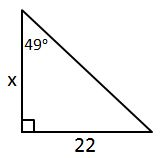#### Question 8 8. In the pictured triangle, ∠A is 58 degrees and ∠C is 63 degrees. If side a is 84 units long, approximately how long is side c?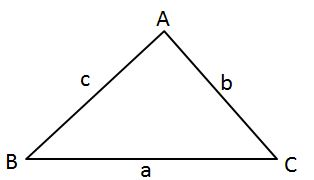### Page 3

#### Question 11 11. In the pictured triangle, ∠A is 98 degrees and ∠B is 12 degrees. If side a is 84 units long, approximately how long is side b?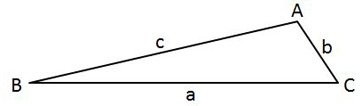### Page 4

#### Question 16 16. In the pictured triangle, what ratio represents the cosine of theta?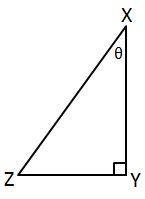#### Question 20 20. In the pictured triangle, side b is 12 and side c is 16. If ∠A is 84 degrees, then approximately how long is side a? Round the value of the cosine to the nearest whole number.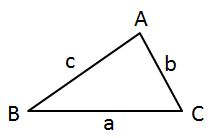### Page 5

#### Question 24 24. Find the value of x, rounded to the nearest degree.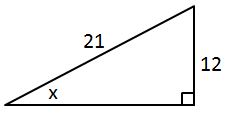#### Question 25 25. In the pictured triangle, ∠A is 81 degrees and ∠B is 67 degrees. If side a is 34 units long, approximately how long is side b?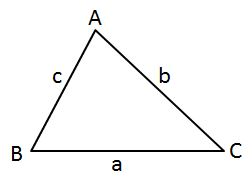### Page 6

#### Question 30 30. In the pictured triangle, side a is 9 and side b is 6. If ∠C is 26 degrees, then approximately how long is side c? Round the value of the cosine to the nearest whole number.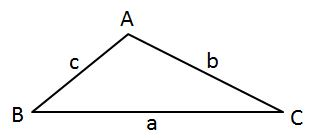#### Big Ideas Math Geometry Chapter 9: Right Triangles and Trigonometry Chapter Exam Instructions

Choose your answers to the questions and click 'Next' to see the next set of questions. You can skip questions if you would like and come back to them later with the yellow "Go To First Skipped Question" button. When you have completed the practice exam, a green submit button will appear. Click it to see your results. Good luck!

Support